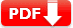# ASVAB Math Practice Test 2021 Free Printable PDF Worksheet

ASVAB Math Practice Test 2021 Free Printable PDF Worksheet: Mathematics Knowledge Question Answers Free Download for Armed Services Vocational Aptitude Battery exam and Armed Forces Qualification Test (AFQT) exam preparation online.

Mathematics Knowledge (MK) tests the ability to solve problems by applying knowledge of mathematical concepts and applications. The problems focus on concepts and algorithms and involve number theory, numeration, algebraic operations and equations, geometry and measurement, and probability. Mathematics Knowledge is one factor that characterizes mathematics comprehension; it also assesses logical thinking.

Actual Exam Content in MK Part

 Test Type Number of Question ASVAB Paper-and-Pencil 25-items CAT-ASVAB Test 16-items

## ASVAB Mathematics Knowledge Practice Test 2021

The following ASVAB Mathematics Knowledge Practice Test is only for reference purposes, it’s not an actual exam test question. You can download our free ASVAB Math Practice Question in PDF (printable and editable), this will help you to make better ASVAB Test Prep offline. You can also use these files (PDF, DOC) as worksheets.

 Name of the Test ASVAB Practice Test | AFQT Practice Test ASVAB Stands for Armed Services Vocational Aptitude Battery AFQT Stands for Armed Forces Qualification Test Test Type Sample Multiple Choice Test Total Questions 25 (twenty-five) Answers and Explanation Available Printable and Editable PDF Available Subject Name Mathematics Knowledge (MK) Purpose Measures knowledge of mathematical concepts and applications

## ASVAB Math Practice Test 2020

Q1. x + 9 = 13, Find x = ?

• A. 3
• B. 4
• C. 5
• D. 13

Isolate the unknown on one side. Subtract 9 => from each side x  = 13 – 9 => x = 4

Q2. 3² + 4(5 – 2) = ?

• A. 21
• B. 36
• C. 39
• D. 40

Perform operations in parentheses first, then exponents, and then the remaining operations.

Q3.  1 \frac14 × 2 \frac12 = ?

• A. 2 \frac12
• B. 2 \frac14
• C. 2\frac34
• D. 3 \frac18

Change the mixed numbers to improper fractions. Carry out the operation by multiplying thet wo numerators and the two denominators.

Q4. The square root of 64 =

• A. 4
• B. −8
• C. 9
• D. −9

−8 ×−8 = 64

Q5. . What is the value of the following expression if x = 2 and y = 4?

2x³y²

• A. 52
• B. 106
• C. 256
• D. 512

Substitute 2 for x and 4 for y into the expression. Multiply out the exponents.

3x² + 3xy + 4y + 2y²
3x² + xy + 9y + y²
−5x² − 2xy − 13y + y²
−3x² + 3xy + y − 4y

• A. 2x + xy + 2y + y²
• B. − 2x − xy + y + y²
• C.  −3x² − 2xy − 3y + y²
• D. −2x² + 5xy + y

Q7. Factor the following expression: y² − 16y + 48

• A. (y − 1)(y +48)
• B. (y − 4)(y − 12)
• C. (y − 4)(y + 4)
• D. (y +1)(y − 16)

each term. In this problem, the fact that the two terms will have a minus sign should lead you to the correct answer, as only one answer has a minus sign in each term. However, not all questions of this type will have that kind of clue. Note that the first term in each factor will be y. Now you need to find two numbers that multiply to 48 and add to 16. Those numbers are 4 and 12. This gives the factors (y − 4)(y − 12)

Q8. Solve for x. 12x + 6 = 8x +10

• A. x = 1
• B. x = 2
• C. x = 4
• D. x = 6

To solve such problems, move all the terms with an unknown to one side of the equal sign and the numbers to the other.

Q9. Solve for the two unknowns.
3y + 3x = 24
6y + 3x = 39

• A. y = 3; x = 5
• B. y = 4; x = 6
• C. y = 5; x = 3
• D. y = 6; x = 2

Set each equation equal to zero. Next, arrange one of the equations so that when it is subtracted from or added to the other equation, one of the terms becomes zero and drops out of the equation. Next, solve for the remaining unknown.

Q10. x¹² / x4

• A. x4
• B. x8
• C. x18
• D. x−16

To divide numbers with exponents, subtract the exponents. In this problem, subtract the 4 from the 12, leaving x8 as the correct answer.

Q11. Solve for y.

y² + 7y = −10

• A. y = −5; y = −2
• B. y = 1; y = −10
• C. y = −2; y = 6
• D. y = 5; y = −1

Set the equation to equal zero:

Q12. Multiply: \frac{6y}{11} × \frac{2}{5x}

• A. 6y + 5x =22
• B. 22y / 5x
• C.  5y/ 22x
• D. 22y / 55x

multiply fractions, multiply the numerators and denominators.

Q13. Divide: \frac{6x}{11} ÷ \frac{2}{5y}

• A. 3y + 11x =2
• B. 15xy / 11
• C.  12/ 22xy
• D. 11y / 25x

To divide fractions, invert the second term and multiply.

Q14. \sqrt27

• A. 3
• B. 4
• C. 5
• D. 2.25

The cube root is the number that, when multiplied by itself three times, results in the answer. In this instance, 3 × 3 × 3 = 27, so the cube root of 27 = 3.

To answer most questions of this type on the ASVAB, you just need to memorize some basic cubes. It would be wise to memorize all the
cubes from 2 to 10, just to prepare for ASVAB test item possibilities

Q15. Solve for g \fracgh =a

• A. ga / h
• B. g =a/h
• C. g = ah
• D. g = a ÷ h

Isolate the variable of interest on one side of the equation. In this problem, you must multiply each side by h, leaving g on the left side of the
equation and h on the right side. Therefore, the answer is g = ah.

Q16. Which of the following is an equilateral triangle?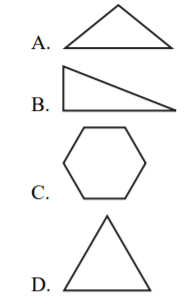An equilateral triangle has three equal sides and angles, so D is the correct answer.

Q17. What is the perimeter of the following rectangle?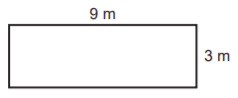• A. 12 m
• B. 18 m
• C. 24 m
• D. 12 m

To find the perimeter of a quadrilateral, add the lengths of the four sides. In this instance, 9 meters + 9 meters + 3 meters + 3 meters + 24

Q18. What is the circumference of the following circle?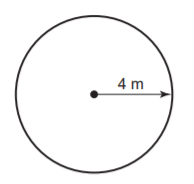• A. 6.56 m
• B. 12.56 m
• C. 25.12 m
• D. 35.12 m

The formula for the circumference of a circle is Πd. The figure gives the radius, which is one-half the length of the diameter. To find the diameter, multiply the radius by 2. In this problem, the diameter is 8 meters. Multiplying 8 by Π gives the correct answer of 25.12 meters.

Q19. In the following equilateral triangle, what is the measure of ∠1?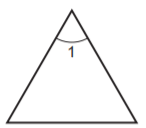• A. 30°
• B. 45°
• C. 60°
• D. 90°

An equilateral triangle has three equal angles. A triangle has a total of 180°, so each angle must be 60°.

20. If ∠1 is 34°, what is the measure of ∠2?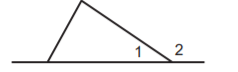• A. 56°
• B. 110°
• C. 146°
• D. 180°

A straight line or straight angle is 180°. So if 1 is 34°, 2 is the difference between 180° and 34°, or 146°.

Q21. In the following right triangle, what is the length of side AB?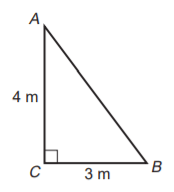• A. 3 m
• B. 5 m
• C. 7 m
• D. 12 m

C is a right angle, so you can employ the Pythagorean Theorem

Q22. What is the area of the following triangle?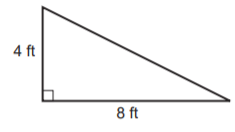• A. 8 ft²
• B. 12 ft²
• C. 15 ft²
• D. 16 ft²

This is a right triangle, so 4 ft is the height of the triangle and 8 ft is the base. The formula for the area of a triangle is ½ bh.

Q23. What is the diameter of the following circle?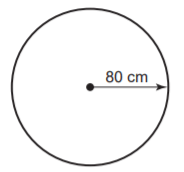• A. 40 cm
• B. 80 cm
• C. 160 cm
• D. 640 cm

The diameter is 2r. r = 80 cm, so the diameter is 160 cm.

Q24. If lines A and B are parallel and are intersected by line C and ∠2 is 30°, what is the measure of ∠7?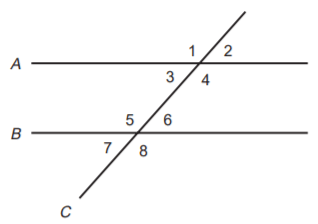• A. 30°
• B. 50°
• C. 112°
• D. 130°

2 and 7 are alternate exterior angles and therefore are equal in measure. So both 2 and 7 are 30°.

Q25. What is the volume of a cylinder with a height of 15 m and a radius of 5 m?

• A. 235.50 m³
• B. 1,177.50 m³
• C. 1,280.25 m³
• D. 1,340.25 m³
 Document Type Download Link Free Editable Doc File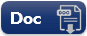Free Printable PDF File